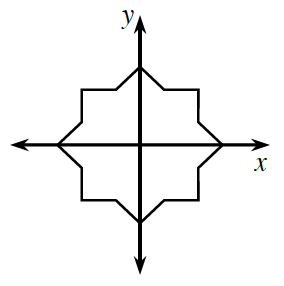### Home > GB8I > Chapter 3 Unit 4 > Lesson INT1: 3.1.3 > Problem3-37

3-37.Examine the figure graphed on the axes at right.

1. What happens when you rotate this figure about the origin $90^{\large \circ }$? $45^{\large \circ }$? $180^{\large \circ }$?

2. By what other angle could the figure at right be rotated so that the shape does not appear to change?

3. What shape will stay the same no matter how many degrees it is rotated?

Use the eTool to rotate the figure. Use what you observe to answer questions (a), (b), and (c).
Click the link at right for the full version of the eTool: